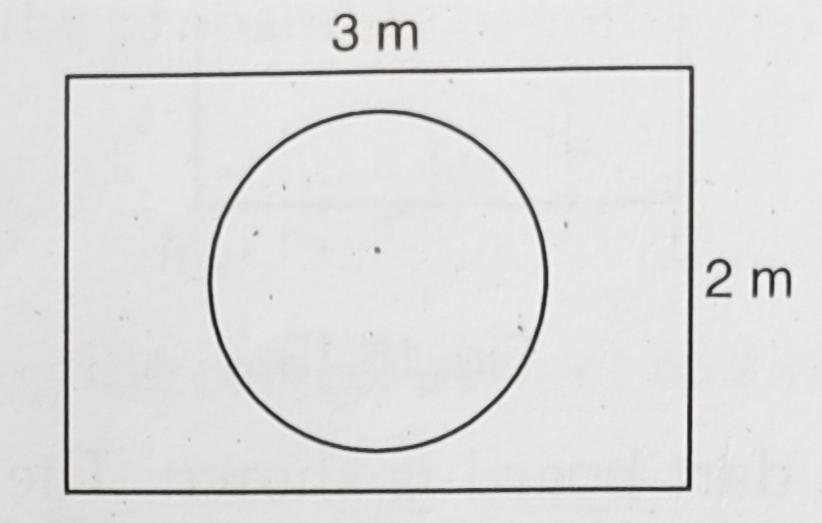" ">

# Suppose you drop a die at random on the rectangular region shown in figure. What is the probability that it will land inside the circle with diameter $1\ m$?"

Given:

Length of the rectangle $=3\ m$

Breadth of the rectangle $=2\ m$

Diameter of the circle $=1\ m$

To do:

We have to find the probability that the tie will land inside the circle.

Solution:

Area of a rectangle of length $l$ and breadth $b$ is $lb$.

This implies,

Area of the rectangle $=3 \times 2=6 \mathrm{~m}^{2}$

Diameter of circle $=1 \mathrm{~m}$

This implies,

Radius $(r)=\frac{1}{2} \mathrm{~m}$

Area of the circle $=\pi r^{2}=\pi \times(\frac{1}{2})^{2}=\frac{1}{4} \pi\ \mathrm{m}^{2}$

Therefore,

Probability that it will land inside the circle $=\frac{Area\ of\ the\ circle}{Area\ of\ the\ rectangle}$

$=\frac{\frac{1}{4} \pi}{6}$

$=\frac{1 \times \pi}{4 \times 6}$

$=\frac{\pi}{24}$

The probability that the tie will land inside the circle is $\frac{\pi}{24}$.

Updated on: 10-Oct-2022

46 Views